My notes on `Linked list` with an implementation in Java.

You can find the complete code for this and other data structures here: data structures and algorithms

A `linked list` is a `data structure` that uses `nodes` that hold both a value and a reference to the `next node`.

Common Operations for a linked list includes:

Operation Complexity
get(indexOfElement) O(n)
insert(index, value) O(n)
find(value) O(n)
delete(value) O(n)

This is the node we will be using: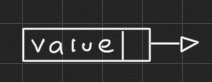In this implementation of the linked list we will hold both a `reference` to the `head` and the `tail` of the list. We will be only accepting objects of classes that implement the `Comparable` interface since that way we can easily compare between two values.

At the beginning the list will look like this: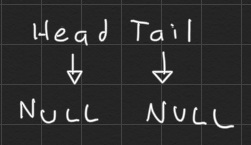If we add an element to the list it will look like this, with both head and tail pointing to the same node.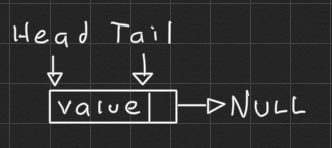To `append` an element at the end of the list we can use the tail reference, add the element as the next element of the tail: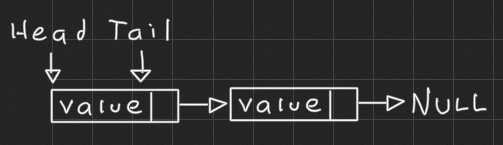Then we update the tail reference: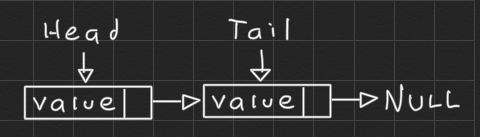For `prepending` an element to the beginning of the list we first create the new element and set the head as the new elements next node: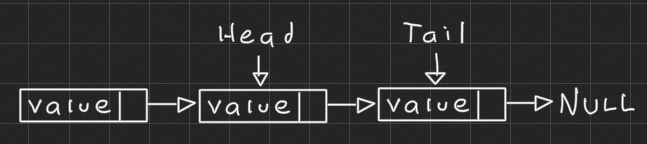And then we update the head referenceTo find a value from an index we just iterate the linked list counting every node.

To find the index of an element using the value we just iterate the linked list until we run out of list, or we find the element.

To remove an element using its value we will use two references, `previous` and `current`, and when current points to the value we want to remove we will use the previous reference to take out of the list the element we want to remove.

Suppose in this case current points to the value we want to remove:We update the next node of previous: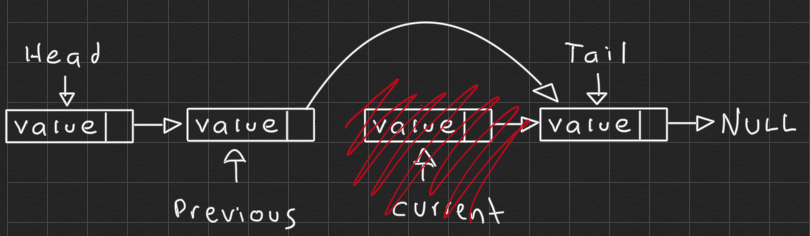Insertion at any index works by getting a reference to the node right before the index where we want to insert, and then updating the next node references.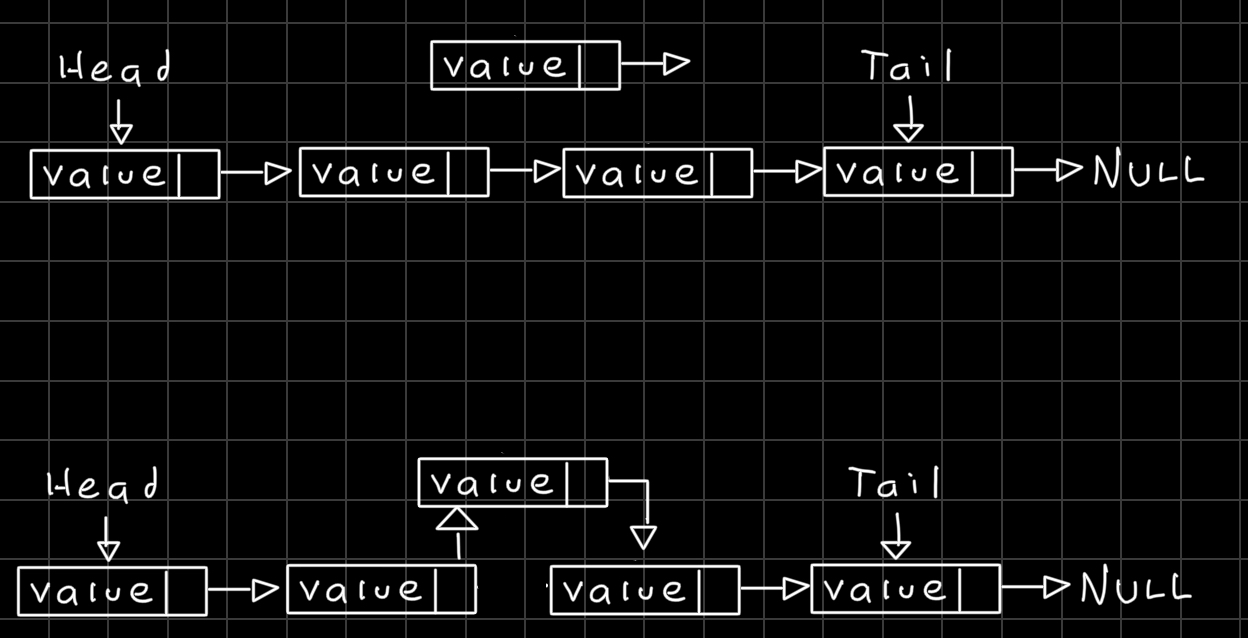Download the complete code for this and other data structures here: data structures and algorithms